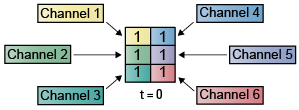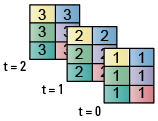## Create Signals for Sample-Based Processing

In sample-based processing, blocks process signals one sample at a time. Each element of the input signal represents one sample in a distinct channel. For example, from a sample-based processing perspective, the following 3-by-2 matrix contains the first sample in each of six independent channels.When you configure a block to perform sample-based processing, the block interprets scalar input as a single-channel signal. Similarly, the block interprets an M-by-N matrix as multichannel signal with M*N independent channels. For example, in sample-based processing, blocks interpret the following sequence of 3-by-2 matrices as a six-channel signal.For more information about the recent changes to frame-based processing, see the Frame-based processing changes section of the DSP System Toolbox™ Release Notes.

This page discusses creating signals for sample-based processing using the Constant block and the Signal From Workspace block. Note that the block receiving this signal implements sample-based processing or frame-based processing on the signal based on the parameters set in the block dialog box.

### Create Signals Using Constant Block

1. Create a new Simulink® model.

2. From the Sources library, click-and-drag a Constant block into the model.

3. From the Sinks library, click-and-drag a Display block into the model.

4. Connect the two blocks.

5. Double-click the Constant block, and set the block parameters as follows:

• Constant value = ```[1 2 3; 4 5 6]```

• Interpret vector parameters as 1–D = Clear this check box

• Sample time = `1`

Based on these parameters, the Constant block outputs a constant, discrete-valued, `2`-by-`3` matrix signal with a sample period of `1` second.

The Constant block's Constant value parameter can be any valid MATLAB® variable or expression that evaluates to a matrix.

6. Save these parameters and close the dialog box by clicking .

7. In the Debug tab of the model toolstrip, select > .

8. Run the model and expand the Display block so you can view the entire signal.

You have now successfully created a six-channel signal with a sample period of 1 second.

To view the model you just created, and to learn how to create a 1–D vector signal from the block diagram you just constructed, continue to the next section.

#### Create an Unoriented Vector Signal

You can create an unoriented vector by modifying the block diagram you constructed in the previous section:

1. To add another signal to your model, copy the block diagram you created in the previous section and paste it below the existing signal in your model.

2. Double-click the Constant1 block, and set the block parameters as follows:

• Constant value = ```[1 2 3 4 5 6]```

• Interpret vector parameters as 1–D = Check this box

• Sample time = `1`

3. Save these parameters and close the dialog box by clicking .

4. Run the model and expand the Display1 block so you can view the entire signal.

Your model should now look similar to the following figure. You can also open this model by typing `ex_usingcnstblksb` at the MATLAB command line.The Constant1 block generates a length-6 unoriented vector signal. This means that the output is not a matrix. However, most nonsource signal processing blocks interpret a length-M unoriented vector as an M-by-1 matrix (column vector).

### Create Signals Using Signal From Workspace Block

This topic discusses how to create a four-channel signal for sample-based processing with a sample period of `1` second using the Signal From Workspace block:

1. Create a new Simulink model.

2. From the Sources library, click-and-drag a Signal From Workspace block into the model.

3. From the Simulink Sinks library, click-and-drag a To Workspace block into the model.

4. Connect the two blocks.

5. Double-click the Signal From Workspace block, and set the block parameters as follows:

• Signal = ```cat(3,[1 -1;0 5],[2 -2;0 5],[3 -3;0 5])```

• Sample time = `1`

• Samples per frame = `1`

• Form output after final data value by = `Setting to zero`

Based on these parameters, the Signal From Workspace block outputs a four-channel signal with a sample period of 1 second. After the block has output the signal, all subsequent outputs have a value of zero. The four channels contain the following values:

• Channel 1: 1, 2, 3, 0, 0,...

• Channel 2: -1, -2, -3, 0, 0,...

• Channel 3: 0, 0, 0, 0, 0,...

• Channel 4: 5, 5, 5, 0, 0,...

6. Save these parameters and close the dialog box by clicking .

7. In the Debug tab of the model toolstrip, select > .

8. Run the model.

The following figure is a graphical representation of the model's behavior during simulation. You can also open the model by typing `ex_usingsfwblksb` at the MATLAB command line.9. At the MATLAB command line, type `yout`.

The following is a portion of the output:

```yout(:,:,1) = 1 -1 0 5 yout(:,:,2) = 2 -2 0 5 yout(:,:,3) = 3 -3 0 5 yout(:,:,4) = 0 0 0 0 ```

You have now successfully created a four-channel signal with sample period of 1 second using the Signal From Workspace block. This signal is used for sample-based processing.

Watch now